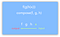# What I Learned Today 💡 July 2, 2017

## Functional Composition: compose() and pipe()

Functions are at the heart of Functional Programming (duh). They are the atomic building block of FP.

As such, a fundamental idea of FP is the “stitching-together” of functions — producing complex functionality by composing small functions that do one thing.

This is function composition. This is achieved by passing the output of one function as the input of another function.

# Raw Composition

Consider the following mathematical functions:

`f(x) = x + 2g(x) = 4x`

You could compose these functions as such:

`f(g(x)) = 4x + 2// org(f(x)) = 4x + 8`

Here’s a programming analog:

`const addTwo = x => x + 2;const multiplyByFour = x => 4 * x;`

Like in the mathematical example above, you could compose them as:

`const composed1 = x => addTwo(multiplyByFour(x));// orconst composed2 = x => multiplyByFour(addTwo(x));`

Here’s a demo:

# Problem

The problem is that composing functions like this doesn’t scale well.
If you have many functions, your composition might end up looking like:

`func1(func2(func3(func4(func5(func6(func7(x)))))))`

Which is absolutely unsightly.

How do we solve this? `compose()` and `pipe()`.

# compose()

`compose()` takes functions as input, connects them such that the data flows from RIGHT to LEFT, then returns this combined function.The LEFT ← RIGHT flow is for matching the mathematical notation.

Here is an implementation:

Here is an example usage:

You can see that the data flowed `LEFT <-- RIGHT`.

`20 <-- multiplyByFour(5) <-- 5 <-- addTwo(3) <-- 3`

This is much better than the raw composition from before. 😊

# pipe()

`pipe()` is nearly identical to `compose()`. The only difference is that `pipe()` moves data in the opposite direction: `LEFT --> RIGHT`.

Here is an implementation. The only difference is that `Array.prototype.reduce` is used instead of `Array.prototype.reduceRight`:

Here is the same example usage. The only difference is that `addTwo` and `multiplyByFour` swapped positions. Again, this is because `pipe()` moves data from `LEFT --> RIGHT`.

Here is a demo:

I personally prefer `pipe()` to `compose()` because I find `LEFT --> RIGHT` data-flow more natural. My English-oriented mind naturally reads that way.

Maybe speakers of right-to-left languages and mathematicians prefer `compose()`.

Opinions expressed in these articles do not reflect those of my employer.

Written by

Written by